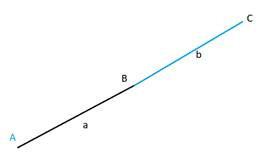# Methods for finding three points are collinear or not; using slope, distance formula or any other method.### Precalculus: Mathematics for Calcu...

6th Edition
Stewart + 5 others
Publisher: Cengage Learning
ISBN: 9780840068071### Precalculus: Mathematics for Calcu...

6th Edition
Stewart + 5 others
Publisher: Cengage Learning
ISBN: 9780840068071

#### Solutions

Chapter 1.10, Problem 78E
To determine

## Methods for finding three points are collinear or not; using slope, distance formula or any other method.

Expert Solution

Using slope: If slope of first two point is same as other two point, then all three points are collinear.

Using Distance Formula: if the sum of distance of first-second point and second-third point is equal to first-third point, then the three points are collinear.

Using Area of triangle: if area enclosed by the three points in 0, then all of them are collinear.

### Explanation of SolutionUsing slope: slope of AB=slope of BC

Using Distance formula: a+b=AC

Area method: ABC=0

### Have a homework question?

Subscribe to bartleby learn! Ask subject matter experts 30 homework questions each month. Plus, you’ll have access to millions of step-by-step textbook answers!